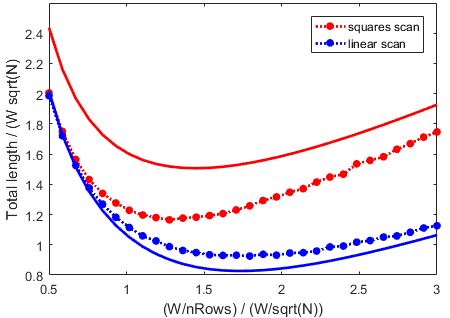Categories

# Approximate TSP Paths

Let’s say you have lots of points, and you want to visit them rapidly. And you’re too lazy to solve the full TSP. How well do different approximations work? That is, how far would you have to travel to visit each point?

Let’s say you have N points that are uniformly randomly distributed in a square with side length W. (It turns out that using a general rectangle is much more complicated, at least for the first step!)

For unordered points, this is basically (N-1)*(expected distance between two random points in a square), yielding $0.5214 N W$.

As a best-case, what is the average optimal TSP path length? They estimate around $0.7 \sqrt{N} W$, but it’s an open question.

Let’s try some rough methods, and estimate their path length. The following image shows examples of the methods:

## Probabilistic Estimates

How about just sorting them by their x-position? Then it will be a zig-zag line moving up and down from left to right. Then we can estimate the average distance between a pair of points. For flexibility, let’s work in a rectangle of width W and height H. First, the x-displacement is roughly exponentially distributed: $p[dx] = \frac{N}{W}\exp\left( \frac{N}{W} dx \right)$, with $E[dx] = W/N$. The y-displacement is the distance between two random points on a line segment of length H, which gives $E[dy] = H/3$. So a decent approximate total distance is $N\sqrt{\left(\frac{W}{N} \right)^2+\left(\frac{H}{3}\right)^2}$. With lots of points, then we have distance $H N/3$, which isn’t great. Better than entirely unordered points, though.

How about breaking it into small squares of width w, and scanning back and forth along the grid of squares? We know the expected length inside a square, but how about the transition? It is an annoying integral, but the expected distance from a random point in one square to the next is nearly 1.0886 w. Then the total scanning distance is the total distance within the squares, and the connecting jumps, which is approximately $N_{squares} * 0.5214 w N_{persquare} + N_{squares}w 1.0886$, which is optimized with $w \approx 1.44 W/\sqrt{N}$, yielding a distance of $1.51 W \sqrt{N}$.

How about scanning back and forth on the image? That is, cutting the image into bands, and sorting by position on that band. Using the result just above, if we have B bands, then $H=W/B$, so distance is $B N/B\sqrt{\left(\frac{W}{N/B} \right)^2+\left(\frac{W}{3B}\right)^2}+B H$. Minimizing this length gives $B=\sqrt{N/3}$, yielding distance $W \sqrt{2N/3} \approx 0.81 \sqrt{N} W$. This is a serious improvement over just y-sorting, and a moderate improvement over the square-clumping.

## Numerical Experiments

Doing math is always fun, but sometimes it’s not quite tied to reality. Let’s run some tests.

With my greedy TSP algorithms, the method that adds the closest point to the end of the path yields about $0.87\sqrt{N} W$, and the greedy loop method has around $0.81 \sqrt{N} W$. That’s pretty good, but quite expensive to compute.

Here’s a plot of the numerical and analytic results. The analytic models were much too pessimistic for the series of squares, and slightly optimistic for the linear scanning. On the bright side, they did a good job estimating the optimal size for the squares and rows.Theory is solid lines, numerical experiments are dots. The x-axis is basically the row width, and the y-axis is the scaled total distance.

The linear scan works just fine! Of course, this is for uniformly distributed random points. If instead we use blue-noise points, everything gets worse, but the linear scan method still works decently.

What about using everyone’s favorite space-filling Hilbert curve? With random points, and high resolution, this results in a total length of about $0.98\sqrt{N} W$. And with non-uniform points, the Hilbert approach is still pretty good, doing about 20% worse than the greedy loop method, where the scan does 30% worse. The Hilbert curve is particularly useful because of its locality-preserving properties.